Anzeige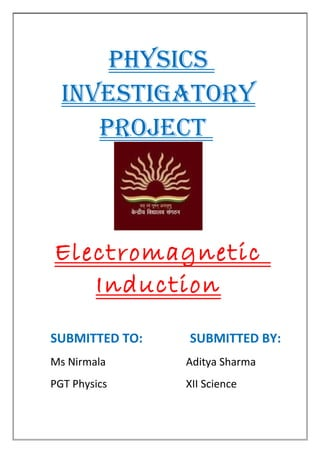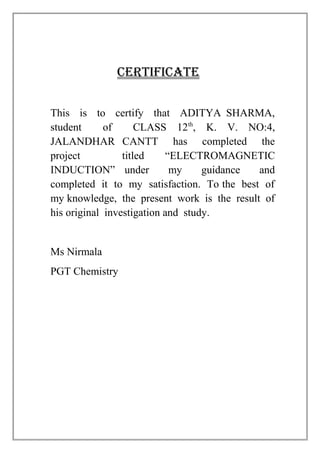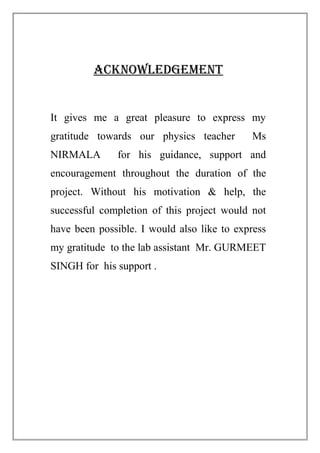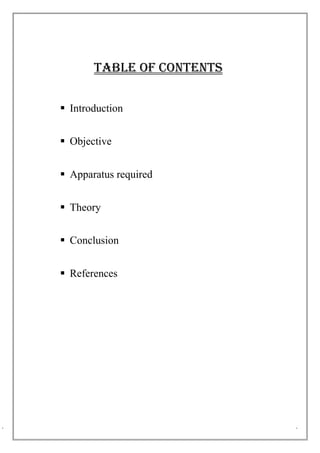Anzeige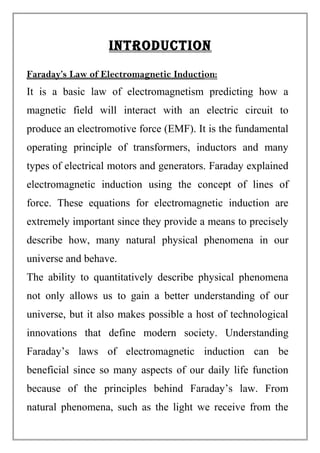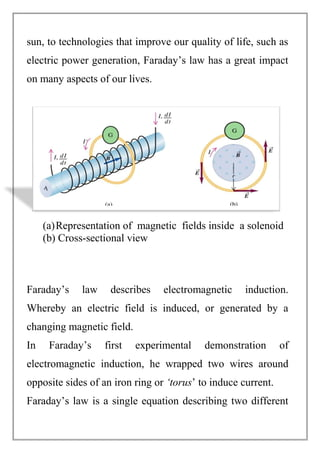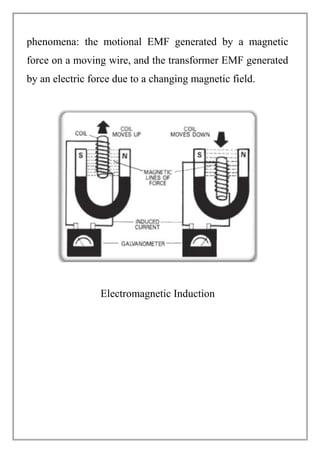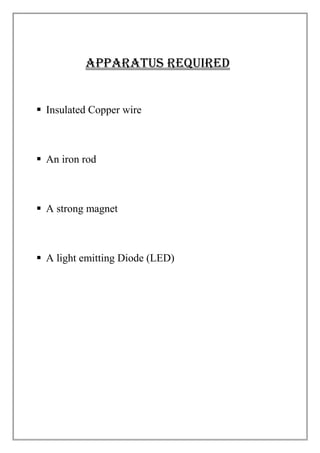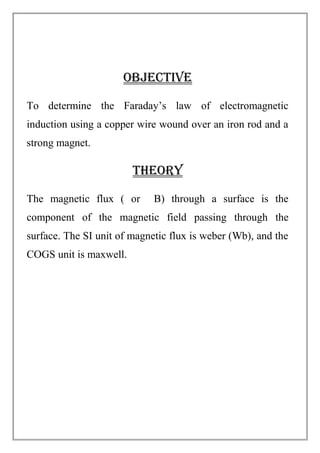Anzeige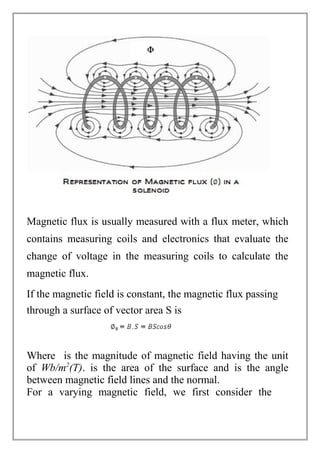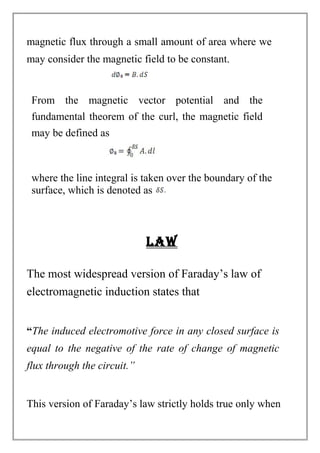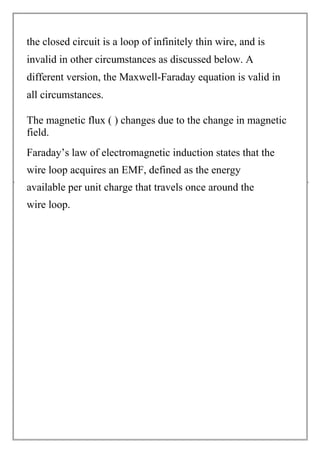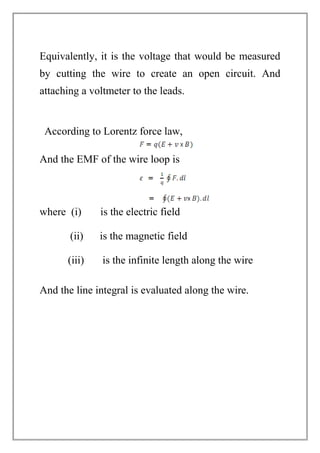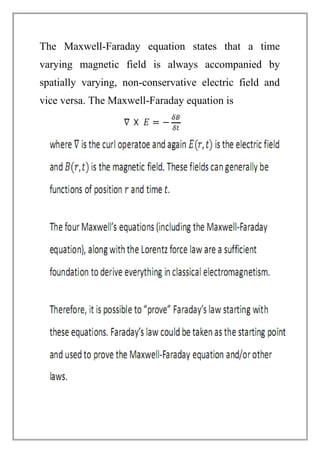Anzeige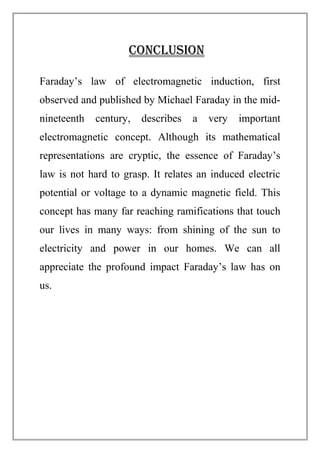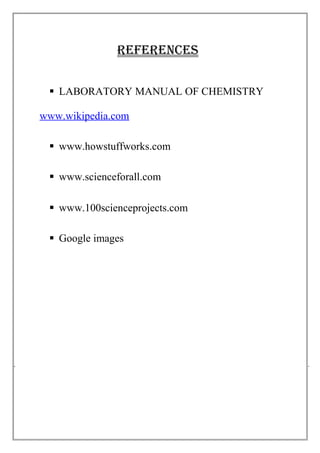Nächste SlideShare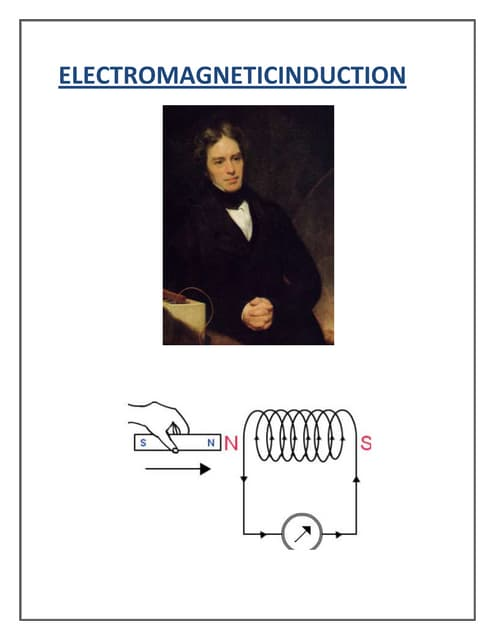Physics project class 12 EMI
1 von 16
Anzeige

### Physics project file on emi

1. PHYSICS INVESTIGATORY PROJECT Electromagnetic Induction SUBMITTED TO: SUBMITTED BY: Ms Nirmala Aditya Sharma PGT Physics XII Science
2. CERTIfICATE This is to certify that ADITYA SHARMA, student of CLASS 12th , K. V. NO:4, JALANDHAR CANTT has completed the project titled “ELECTROMAGNETIC INDUCTION” under my guidance and completed it to my satisfaction. To the best of my knowledge, the present work is the result of his original investigation and study. Ms Nirmala PGT Chemistry
3. ACKNOWLEDGEMENT It gives me a great pleasure to express my gratitude towards our physics teacher Ms NIRMALA for his guidance, support and encouragement throughout the duration of the project. Without his motivation & help, the successful completion of this project would not have been possible. I would also like to express my gratitude to the lab assistant Mr. GURMEET SINGH for his support .
4. TABLE Of CONTENTS  Introduction  Objective  Apparatus required  Theory  Conclusion  References
5. INTRODUCTION Faraday’s Law of Electromagnetic Induction: It is a basic law of electromagnetism predicting how a magnetic field will interact with an electric circuit to produce an electromotive force (EMF). It is the fundamental operating principle of transformers, inductors and many types of electrical motors and generators. Faraday explained electromagnetic induction using the concept of lines of force. These equations for electromagnetic induction are extremely important since they provide a means to precisely describe how, many natural physical phenomena in our universe and behave. The ability to quantitatively describe physical phenomena not only allows us to gain a better understanding of our universe, but it also makes possible a host of technological innovations that define modern society. Understanding Faraday’s laws of electromagnetic induction can be beneficial since so many aspects of our daily life function because of the principles behind Faraday’s law. From natural phenomena, such as the light we receive from the
6. sun, to technologies that improve our quality of life, such as electric power generation, Faraday’s law has a great impact on many aspects of our lives. (a)Representation of magnetic fields inside a solenoid (b) Cross-sectional view Faraday’s law describes electromagnetic induction. Whereby an electric field is induced, or generated by a changing magnetic field. In Faraday’s first experimental demonstration of electromagnetic induction, he wrapped two wires around opposite sides of an iron ring or ‘torus’ to induce current. Faraday’s law is a single equation describing two different
7. phenomena: the motional EMF generated by a magnetic force on a moving wire, and the transformer EMF generated by an electric force due to a changing magnetic field. Electromagnetic Induction
8. APPARATUS REQUIRED  Insulated Copper wire  An iron rod  A strong magnet  A light emitting Diode (LED)
9. OBJECTIVE To determine the Faraday’s law of electromagnetic induction using a copper wire wound over an iron rod and a strong magnet. THEORY The magnetic flux ( or B) through a surface is the component of the magnetic field passing through the surface. The SI unit of magnetic flux is weber (Wb), and the COGS unit is maxwell.
10. Magnetic flux is usually measured with a flux meter, which contains measuring coils and electronics that evaluate the change of voltage in the measuring coils to calculate the magnetic flux. If the magnetic field is constant, the magnetic flux passing through a surface of vector area S is Where is the magnitude of magnetic field having the unit of Wb/m2 (T). is the area of the surface and is the angle between magnetic field lines and the normal. For a varying magnetic field, we first consider the
11. magnetic flux through a small amount of area where we may consider the magnetic field to be constant. From the magnetic vector potential and the fundamental theorem of the curl, the magnetic field may be defined as where the line integral is taken over the boundary of the surface, which is denoted as LAW The most widespread version of Faraday’s law of electromagnetic induction states that “The induced electromotive force in any closed surface is equal to the negative of the rate of change of magnetic flux through the circuit.” This version of Faraday’s law strictly holds true only when
12. the closed circuit is a loop of infinitely thin wire, and is invalid in other circumstances as discussed below. A different version, the Maxwell-Faraday equation is valid in all circumstances. The magnetic flux ( ) changes due to the change in magnetic field. Faraday’s law of electromagnetic induction states that the wire loop acquires an EMF, defined as the energy available per unit charge that travels once around the wire loop.
13. Equivalently, it is the voltage that would be measured by cutting the wire to create an open circuit. And attaching a voltmeter to the leads. According to Lorentz force law, And the EMF of the wire loop is where (i) is the electric field (ii) is the magnetic field (iii) is the infinite length along the wire And the line integral is evaluated along the wire.
14. The Maxwell-Faraday equation states that a time varying magnetic field is always accompanied by spatially varying, non-conservative electric field and vice versa. The Maxwell-Faraday equation is
15. CONCLUSION Faraday’s law of electromagnetic induction, first observed and published by Michael Faraday in the mid- nineteenth century, describes a very important electromagnetic concept. Although its mathematical representations are cryptic, the essence of Faraday’s law is not hard to grasp. It relates an induced electric potential or voltage to a dynamic magnetic field. This concept has many far reaching ramifications that touch our lives in many ways: from shining of the sun to electricity and power in our homes. We can all appreciate the profound impact Faraday’s law has on us.
16. REFERENCES  LABORATORY MANUAL OF CHEMISTRY www.wikipedia.com  www.howstuffworks.com  www.scienceforall.com  www.100scienceprojects.com  Google images
Anzeige